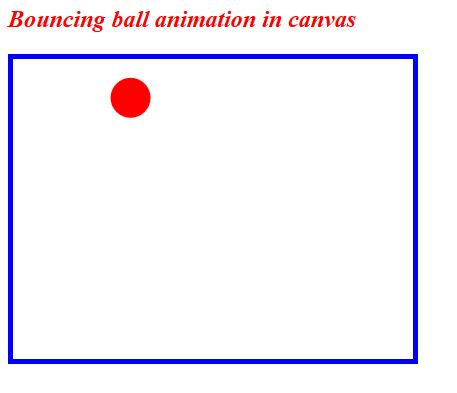# Bouncing Ball in Canvas in HTML5

### Bouncing ball

We can bounce the ball using canvas HTML5

```
<h2 style="color:red;"><b><i>Bouncing ball animation in canvas</i></b></h2>
<canvas id="mycanvas" width="400" height="300" style="border:5px solid blue;"></canvas>
<script>
var canvas = document.getElementById('mycanvas');
var ctx = canvas.getContext('2d');

var p = { x: 25, y: 25 };
var velo = 3,
corner = 50,
var ball = { x: p.x, y: p.y };
var moveX = Math.cos(Math.PI / 180 * corner) * velo;
var moveY = Math.sin(Math.PI / 180 * corner) * velo;

function DrawMe() {
ctx.clearRect(0, 0, 400, 300);

if (ball.x > canvas.width - rad || ball.x < rad) moveX = -moveX;
if (ball.y > canvas.height - rad || ball.y < rad) moveY = -moveY;

ball.x += moveX;
ball.y += moveY;

ctx.beginPath();
ctx.fillStyle = 'red';
ctx.arc(ball.x, ball.y, rad, 0, Math.PI * 2, false);
ctx.fill();
ctx.closePath();
}
setInterval(DrawMe, 10);

</script>
```

• In the above code snippet we have defined the animation of the ball bouncing in the canvas element
• We are having the canvas element as with the id as `"mycanvas" `, width as `400` and height as `300` and we are having the style as` border `as 5 px solid blue color
• `var canvas = document.getElementById('mycanvas');` - It defines the id as "mycanvas"
• v`ar ctx = canvas.getContext('2d');` - It defines the context to the canvas element
• `var p = { x: 25, y: 25 };` - It defines we are declaring var as p to x as 25 and y as 25 which defines the xpositions and y positions
• `var velo = 3, corner = 50, rad = 20;` - It defines the velo as 3 corner, 50 and rad as 20
• `velo` - It defines the different shapes in the canvas
• `corner`- It defines the rotation of the ball animation
• `rad`- It defines the size of the ball
• var `ball x=p.x`
• var `ball y= p.y`
• `var moveX = Math.cos(Math.PI / 180 * corner) * velo;` - It defines the moveX with the given values
• `var moveY = Math.sin(Math.PI / 180 * corner) * velo;` - It defines the moveY with the given values
• In the nextline we are having function Drawme(), in the function drawme we are defining the values as
• `ctx.clearRect(0, 0, 400, 300);` - It defines the clear rect as` (x, y, width, height)` which sets the position n to the center
• In the nextline we are checking with if condition as
• `if (ball.x > canvas.width - rad || ball.x < rad) moveX = -moveX;` - it defines as if the ball.X greater than canvas width(400)-rad(20)||ball.xlessthan rad(20) is equal to -movX
• `if (ball.y > canvas.height - rad || ball.y < rad) moveY = -moveY;` - it defines as if the ball.Y greater than canvas height(400)-rad(20)||ball.Ylessthan rad(20) is equal to -movY
• `ball.x += moveX;` - I defines the ball.X to the moveX
• `ball.y += moveY;` - It defines the ball.Y to the moveY
• In the nextline we are defining the ball with color, shape,size with the given values
• `setinterval (drawme, 10)` is a function which calls the function (Drawme) with the speed as 10

outputViews: 12301 | Post Order: 116